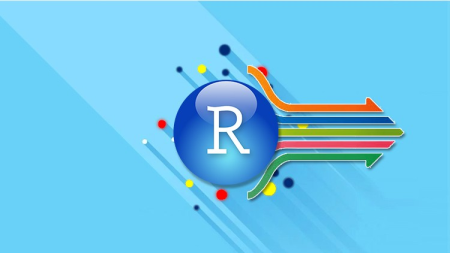# R Programming With Statistics For Data Science

#### Category: Tutorial

Posted on 2021-09-03, by raymanhero.

DescriptionR programming with Statistics for Data science
h264, yuv420p, 1280x720 |ENGLISH, aac, 48000 Hz, 2 channels, s16 | 8h 03 mn | 3.9 GB
Instructors: Hands-On System

======

No prior knowledge of programming is required.

Learn Hands-On applied statistics and data manipulation using real case studies What you'll learn
Learn R programming from scratch
Use of R Studio
Principles of programming
Concept of vectors in R
Data types in R
Know the use of while and for
Build and use matrices in R
Use matrix function, learn rbind and cbind
Install packages in R
Understand the Normal distribution
Practice working with statistical data in R
R functions
Requirements
Just a basic knowledge of computer applications is enough for this course
Description
R is most popular and the leading open source language in data science and statistics. Today, R language is the choice for most data science professionals in every industry and academics.
This course is thoroughly described R programming, Statistics and Data Science for beginners using real life examples.
Let's parse that.
This course does not require a prior quantitative or mathematics background. It starts fundamental concepts of R programming, introducing basic concepts such as the mean, median etc and eventually covers all aspects of an analytics (or) data science career from analyzing and preparing raw data to visualizing your findings.
This course is an introduction to Data Science and Statistics using the R programming language. It covers both the theoretical aspects of Statistical concepts and the practical implementation using R.
Real life examples: Every concept is explained with the help of examples, case studies and source code in R wherever necessary.
Course material in the form for articles include in this program
Data Analysis with R: Datatype and Data structures in R, Vectors, Arrays, Matrices, Lists, Data Frames, Reading data from files, Aggregating, Sorting & Merging Data Frames.
Linear Regression: Regression, Simple Linear Regression in Excel, Simple Linear Regression in R, Multiple Linear Regression in R, Categorical variables in regression, Robust regression, Parsing regression diagnostic plots
Descriptive Statistics: Mean, Median, Mode, Standard Deviation, Frequency Distributions,
Inferential Statistics: Hypothesis testing, Test statistic, Test of significance.
Who this course is for:
Anyone who want to explore R programming
This course is designed for Beginner to professional

Nitroflare
Rapidgator
`https://rapidgator.net/file/c97e8853d486eb470e9867d96f99335b/3XblNswA__R_programm.part1.rar.htmlhttps://rapidgator.net/file/3dcabf54c3ae3631c3ef56e65e0b3271/3XblNswA__R_programm.part2.rar.htmlhttps://rapidgator.net/file/d7ec1a76b8e26cb718c4c446c97c51c8/3XblNswA__R_programm.part3.rar.htmlhttps://rapidgator.net/file/4a01cfff8be0350e2d9488568e6bd0fe/3XblNswA__R_programm.part4.rar.html`

5710 dl's @ 3855 KB/s
6043 dl's @ 2123 KB/s
7225 dl's @ 2342 KB/s

Search More...
R Programming With Statistics For Data ScienceRelated Archive Books

Archive Books related to "R Programming With Statistics For Data Science":

Related Books